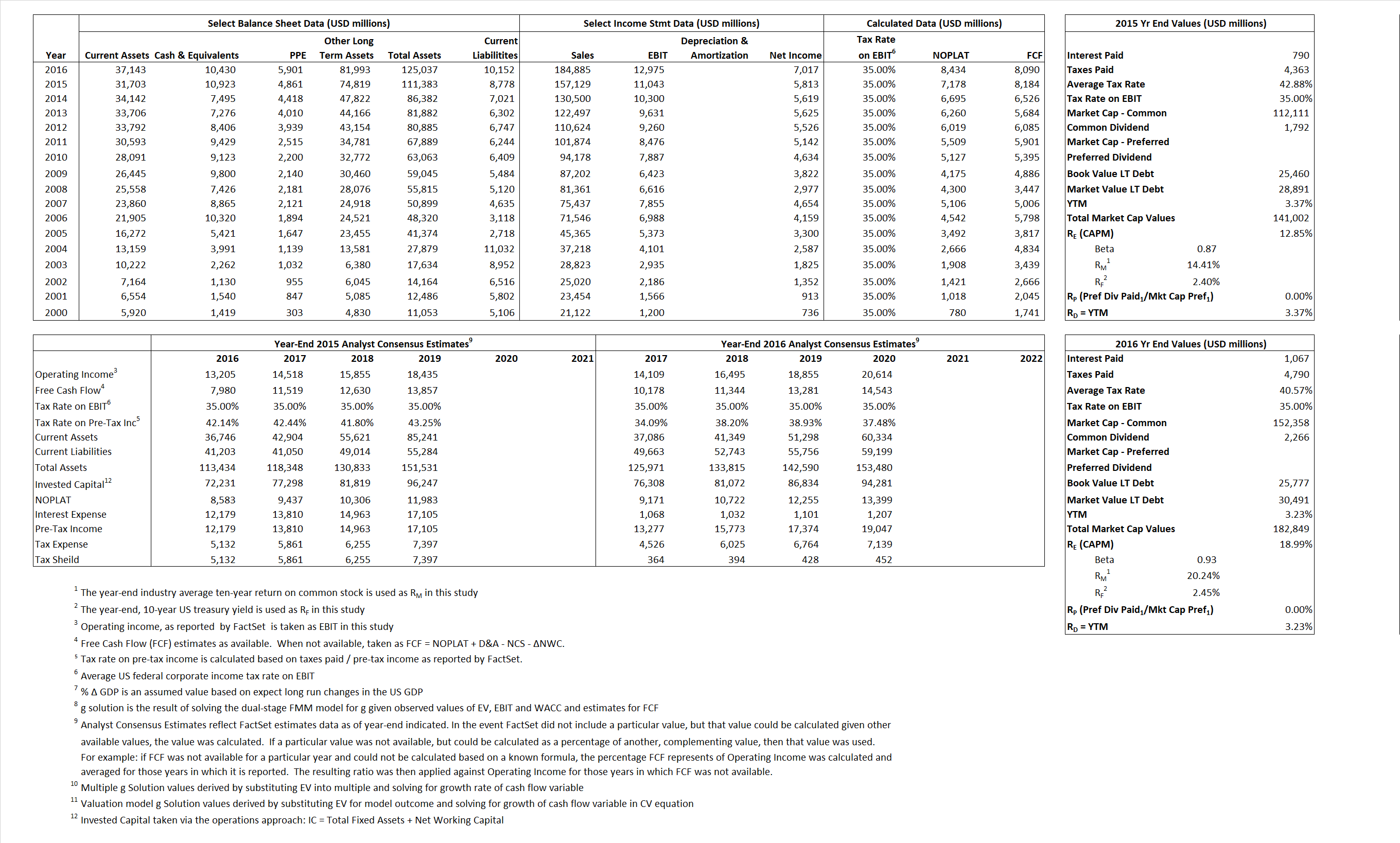# UnitedHealth Group Incorporated

## Analyst Listing

The following analysts provide coverage for the subject firm as of May 2016:

 Broker Analyst Analyst Email Leerink Partners Ana Gupte ana.gupte@leerink.com CRT Capital Group Brian Wright bwright@sterneageecrt.com Susquehanna Financial Group Chris Rigg chris.rigg@sig.com Cowen & Company Christine Arnold christine.arnold@cowen.com Jefferies David Windley dwindley@jefferies.com RBC Capital Markets Frank G. Morgan frank.morgan@rbccm.com Cantor Fitzgerald Joseph D. France jfrance@cantor.com Raymond James Michael J. Baker michael.baker@raymondjames.com Oppenheimer Michael Wiederhorn michael.wiederhorn@opco.com Wells Fargo Securities Peter Costa peter.costa@wellsfargo.com Wedbush Securities Sarah James sarah.james@wedbush.com Credit Suisse Scott Fidel scott.fidel@credit-suisse.com Piper Jaffray Sean W. Wieland sean.w.wieland@pjc.com Mizuho Securities USA Sheryl R. Skolnick sheryl.skolnick@us.mizuho-sc.com FBR Capital Markets & Co Steven Halper shalper@fbr.com Stifel Nicolaus Thomas A. Carroll tacarroll@stifel.com

## Primary Input Data## Derived Input Data

### Equational Form

Net Operating Profit Less Adjusted Taxes NOPLAT 7,178 8,434$NOPLAT\, =\, EBIT\, x\, (1 \,-\, Avg \,\,Tax\,\, Rate\,\, on\,\, EBIT)$
Free Cash Flow FCF 8,184 8,090$FCF\,=NOPLAT\,+\,Non-Cash\,Expenses-\Delta NWC\,-\,NCS$
Tax Shield TS 339 433$TS\,=\,Interest\,\,Paid\,\,x\,\, Avg \,\,Tax\,\,Rate\,\, on\,\, Pre-Tax\,\, Income$
Invested Capital IC 102,605 114,885$IC\,=\,Fixed\,\,Operating\,\,Assets\,\,+\,\,Net\,\, Working\,\, Capital$
Return on Invested Capital ROIC 7.00% 7.34%$ROIC\,=\,\frac { NOPLAT }{ IC }$
Net Investment NetInv 23,244 12,280$NetInv\,=\,{ {IC}_{1}}-{{IC}_{0}}+Depreciation$
Investment Rate IR 323.83% 145.61%$IR\,=\,\frac {NetInv}{NOPLAT}$
Weighted Average Cost of Capital
WACCMarket 10.61% 16.15%$WACC\,=\,\frac { E }{ V } { R }_{ E }\,+\,\frac { P }{ V } { R }_{ P }\,+\,\frac { D }{ V } { R }_{ D }\left( 1- Avg\,\, Tax\,\,Rate\,\,on\,\,Pre-Tax\,\,Income \right)$
WACCBook  6.94%  7.30%
Enterprise value
EVMarket 130,079 172,419$EV\,=\,Market\,\,Cap\,\,Equity\,+\,\,Long\,\,Term\,\,Debt\,-\,Cash$
EVBook  127,141  167,705
Long-Run Growth
g = IR x ROIC
22.65% 10.69% Long-run growth rates of the income variable are used in the Continuing Value portion of the valuation models.
g = %$\Delta$ GDP  2.50%  2.50%
Margin from Operations M 7.03% 7.02%$M\,\,=\,\,\frac{EBIT}{SALES}$
Depreciation/Amortization Rate D 0.00% 0.00%$D\,\,=\,\,\frac{D+A}{EBITDA}$

## Valuation Multiple Outcomes

The outcomes presented in this study are the result of original input data, derived data, and synthesized inputs.

### model g solution

12/31/2015 12/31/2016 12/31/2015 12/31/2016 12/31/2015 12/31/2016

EV/SALES$\frac {EV}{Sales} \,= \,\frac{ROIC\, -\, g}{ROIC\,(WACC\,-\,g)}\,(1\,-\,T)\,(M)$

0.83  0.93  24.11%  33.37%  20.03%  21.23%

EV/EBITDA$\frac {EV}{EBITDA} \,= \,\frac{ROIC\, -\, g}{ROIC\,(WACC\,-\,g)}\,(1\,-\,T)\,(1\,-\,D)$

11.78 13.29 24.11%  33.37% 20.03% 21.23%

EV/NOPLAT$\frac {EV}{NOPLAT} \,= \,\frac{ROIC\, -\, g}{ROIC\,(WACC\,-\,g)}$

18.12 20.44 24.11%  33.37% 20.03% 21.23%

EV/FCFOPS$\frac {EV}{FCF_{OPS}} \,= \,\frac{ROIC\, -\, g}{ROIC\,(WACC\,-\,g)}\,(1\,-\,T)$

15.89 21.31 24.11%  33.37% 20.03% 21.23%

EV/EBIT$\frac {EV}{EBIT} \,= \,\frac{ROIC\, -\, g}{ROIC\,(WACC\,-\,g)}\,(1\,-\,T)$

11.78 13.29 24.11%  33.37% 20.03% 21.23%

EV/IC$\frac {EV}{IC} \,= \,\frac{ROIC\, -\, g}{WACC\,-\,g}$

1.27 1.50 24.11%  33.37% 20.03% 21.23%